# Dividing Decimals by Whole Numbers Lesson Plan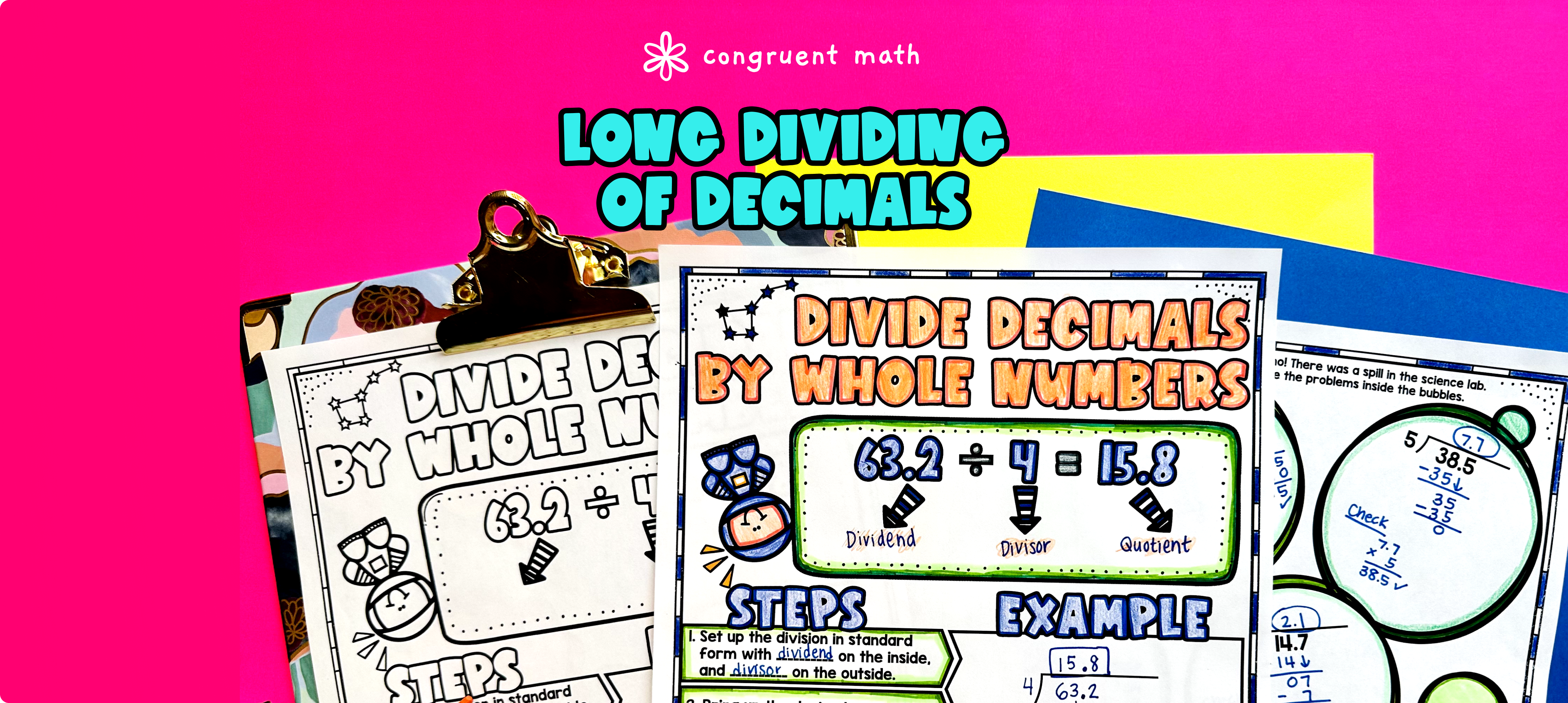Pin This

## Overview

Ever wondered how to teach dividing decimals by whole numbers in an engaging way to your fifth and sixth grade students?

In this lesson plan, students will learn about decimal long division and its real-life applications. Through artistic and interactive guided notes, checks for understanding, practice worksheets, and a real-life application activity, students will gain a comprehensive understanding of dividing decimals by whole numbers.

The lesson begins with guided notes that introduce the topic, including the terms dividend and divisor and the steps for decimal long division. These structured notes help students understand the steps of dividing decimals and provide examples and checks for understanding to ensure they are on the right track.

Next, students will engage in practice worksheets and a color by code activity to reinforce their understanding of dividing decimals by whole numbers. These activities provide hands-on practice and a fun way for students to apply their knowledge.

Finally, the lesson culminates with a real-life application activity where students will read and write about dividing decimals as applied to planning a road trip. This activity helps students see the relevance of the topic in everyday life and encourages critical thinking.

Overall, this lesson plan provides a comprehensive and engaging approach to teaching dividing decimals by whole numbers. Students will not only learn the concept but also apply it to real-world situations, enhancing their understanding and retention of the topic.

## Get the Lesson Materials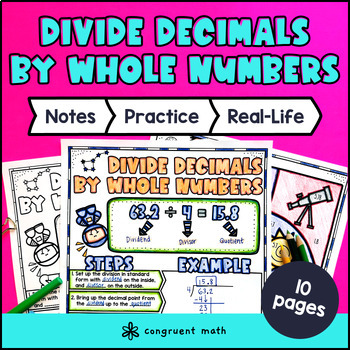\$4.25

## Learning Objectives

After this lesson, students will be able to:

• Define and identify the terms dividend, divisor, and quotient in a division problem involving decimals and whole numbers

• Use the steps of decimal long division to accurately solve division problems involving decimals and whole numbers

• Apply the concept of dividing decimals by whole numbers to real-life situations, such as planning a road trip

## Prerequisites

Before this lesson, students should be familiar with:

• Basic multiplication and division skills of whole numbers

• Understanding of place value and the relationship between whole numbers and decimals

## Key Vocabulary

• Dividing decimals

• Whole numbers

• Decimal long division

• Dividend

• Divisor

• Quotient

• Real-life application

## Procedure

### Introduction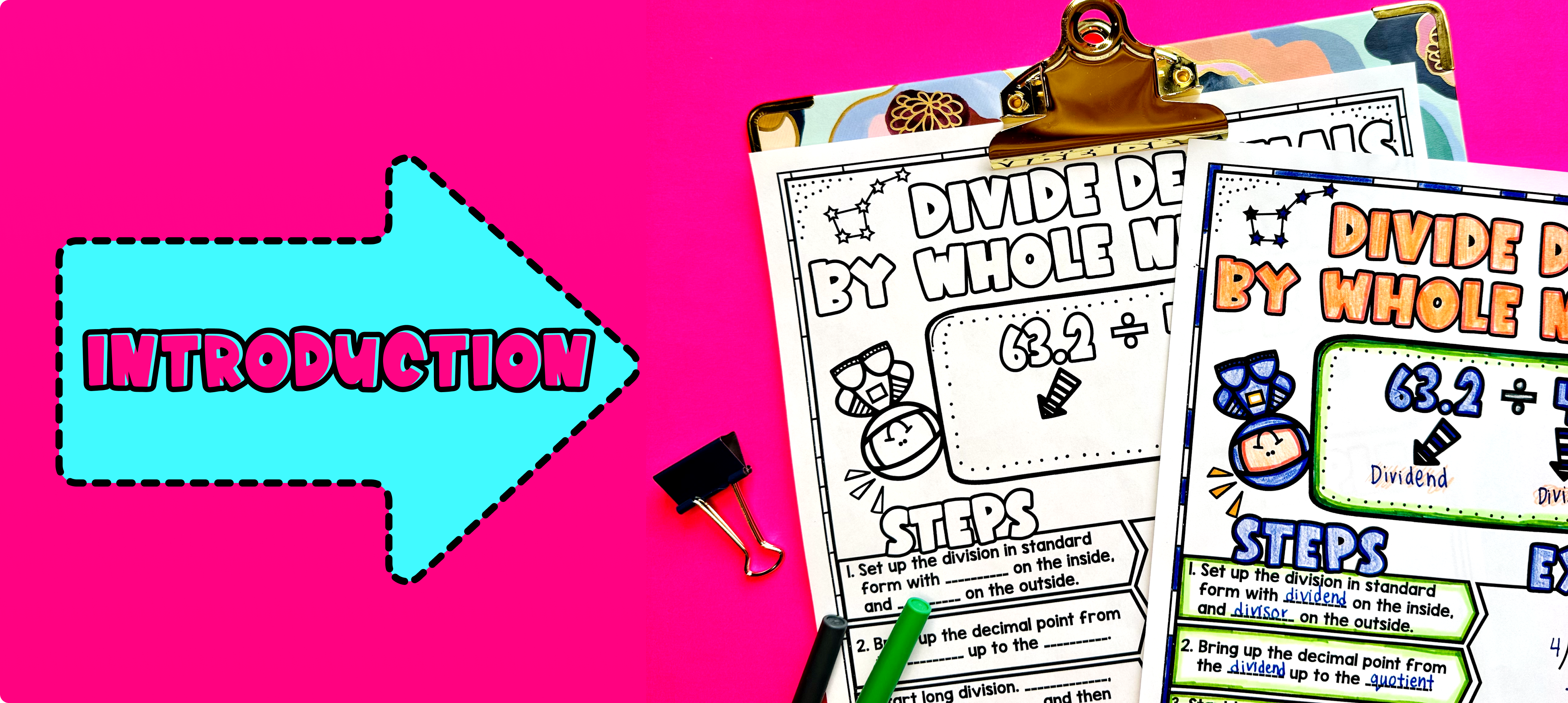Pin This

As a hook, ask students why it is important to be able to divide decimals by whole numbers. This question will help engage students and activate their prior knowledge. Refer to the last page of the guided notes, specifically the real-life application section about planning a road trip, as well as the FAQs below for ideas.

Use the first page of the guided notes to introduce the concept of dividing decimals by whole numbers. Explain to students that in this lesson, they will learn how to divide decimals by whole numbers using decimal long division. Walk through the key points of the topic of the guided notes, such as identifying the dividend and divisor, and walk through the steps required for decimal long division.

Based on student responses and understanding, reteach any concepts or steps that students need extra help with. If your class has a wide range of proficiency levels, you can pull out students for reteaching, and have more advanced students begin work on the practice exercises.

### Practice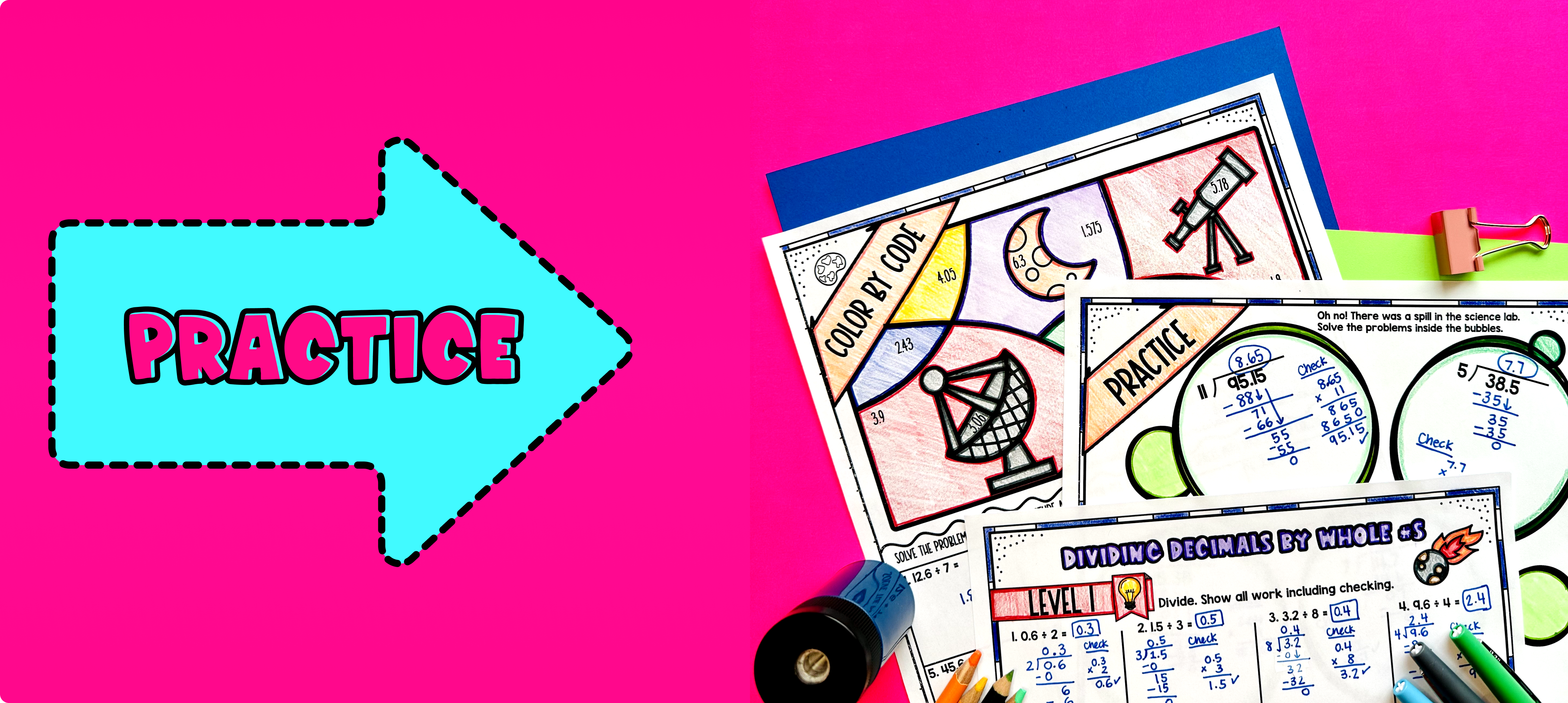Pin This

Have students practice dividing decimals by whole numbers using the maze activity provided in the resource. Walk around the classroom to answer any questions that students may have while they are working on the worksheets.

Fast finishers can engage in the color by code activity included in the resource for extra practice. This can also be assigned as homework for students who need additional practice or for those who finish the worksheets early.

### Real-Life Application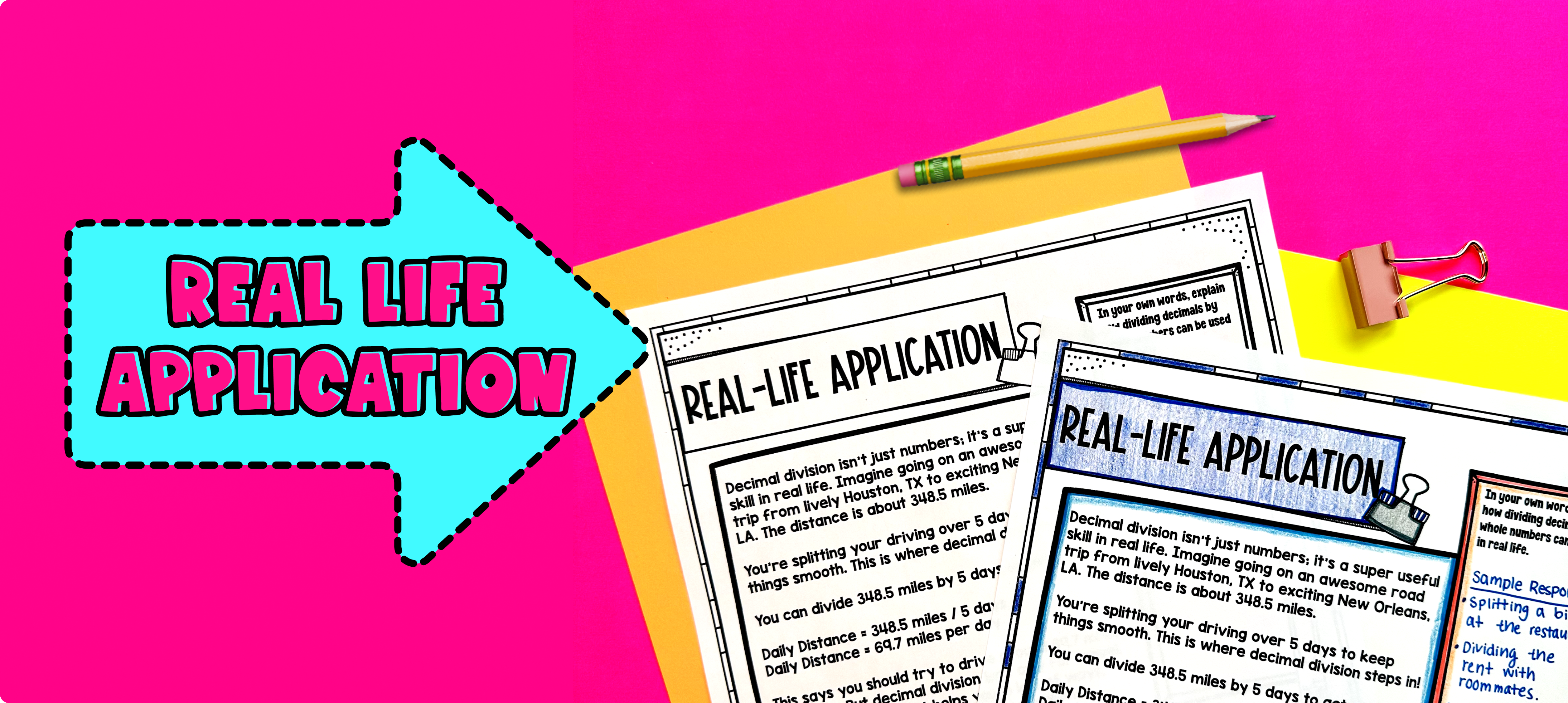Pin This

Bring the class back together, and introduce the concept of dividing decimals by whole numbers in a real-life situation. Explain that understanding how to divide decimals by whole numbers can be helpful when planning a road trip. Use the example on page 5 of the guided notes resource to start the discussion and encourage student reflection on this topic.

Ask students to imagine that they and their family are going on a road trip. Discuss with the class some of the planning that would be involved in this situation. Prompt them with questions such as:

• How would you calculate the distance you need to travel in total? How do you split up the distance over multiple days?

• What if you wanted to know how many tanks of gas you would need for the trip?

Explain to students that dividing decimals by whole numbers can help answer these types of questions. When dividing decimals by whole numbers, the quotient (answer) will often be a decimal. This allows us to calculate measurements, quantities, and costs more precisely.

Refer to the FAQ or discuss other real-world scenarios in which dividing decimals by whole numbers could be helpful. This will help students understand the practical applications of this mathematical concept and its relevance in their everyday lives.

## Extensions

If you're looking for additional print practice to reinforce the concept of dividing decimals by whole numbers, try these Doodle Math activities. They provide a fun and interactive way for students to practice their skills. Each activity includes multiple levels of practice, making it easy to differentiate for your students' needs.

Here are some Doodle Math activities to try: